## 17.2 Models of social situations

The basic models of agents and environments discussed in the previous chapter (see Chapter 16 on Dynamic simulations) were typically developed for individuals. For instance, the framework of reinforcement learning (RL, or the more general class of Markov Decision Processes, MDPs) allows an agent to learn a strategy that maximizes some reward in a stable environment. The options in this environment may be stochastic (e.g., a multi-armed bandit, MAB). Provided that the agent has sufficient time to explore all options, the agent is guaranteed to find the best option. However, if fundamental aspects of the environment change (e.g., by adding options or changing their reward functions), a learning agent may no longer converge on the best option.

Social situations typically change an environment in several ways. Depending on the details of the agent-environment interaction (e.g., how are rewards distributed), they may introduce competition between agents, opportunities for cooperation, and new types of information and learning. For instance, the success of any particular agent strategy can crucially depend on the strategies of other agents. Thus, allowing for other agents questions many results that hold for individual situations and calls for additional types of models and modeling.

In this chapter, we introduce three basic paradigms of modeling social situations:

• games (Section 17.2.1)
• social learning (Section 17.2.2)
• social networks (Section 17.2.3)

### 17.2.1 Games

The scientific discipline of game theory has little resemblance to children’s games, but instead studies mathematical models of strategic interactions among rational decision-makers (see Wikipedia). In a similar vein, the Stanford Encyclopedia of Philosophy defines game theory as

… the study of the ways in which interacting choices of economic agents
produce outcomes with respect to the preferences (or utilities) of those agents,
where the outcomes in question might have been intended by none of the agents.

Ross (2019)

The article immediately adds that the meaning of this definition requires an understanding of the italicized concepts (and provides these details in the corresponding article). For us, it is interesting that games are expressed in terms of economic exchanges, involving agents that pursue their goals, but may obtain outcomes that also depend on the goals and actions of other agents.

We can define a game as a payoff matrix that shows the options and corresponding rewards for its players (in terms of points gained or lost for each combination of outcomes).

One of the simplest possible games is illustrated by Table 17.1. In the matching pennies (MP) game, two players each select one side of a coin (i.e., heads H, or tails T). One player wins if both sides match, the other player wins whenever both sides differ. The payoff matrix shown in Table 17.1 illustrates the options of Player 1 as rows and the first payoff value in each cell, whereas the options of Player 2 are shown as columns and the second payoff values. Thus, Player 1 wins a point ($$+1$$) and Player 2 loses a point ($$-1$$) whenever both players selected the same side of their coins (“HH” or “TT”). Player 2 wins a point ($$+1$$) and Player 1 loses a point ($$-1$$) whenever both players selected different sides of their coins (“HT” or “TH”).

Table 17.1: The payoff matrix of the matching pennies game.
Options H T
H $$(+1, -1)$$ $$(-1, +1)$$
T $$(-1, +1)$$ $$(+1, -1)$$

Winning a game can correspond to maximizing one’s individual reward in a game or to gaining more reward than other players of the same game. As the outcome of any single game may be highly dependent on chance factors, the success of a game-playing strategy is typically assessed over repeated instances of the same game. The goal of maximizing one’s own rewards can create dilemmas when contrasting different levels of aggregation (e.g., single vs. repeated games, or individual vs. population gains).

Games can be classified into types by comparing the players’ reward functions. The most important types of games are:

1. zero-sum or pure competitive games: Some player’s wins correspond to another player’s losses, so that the total of all rewards add up to zero (0).

2. common interest, identical or symmetrical games: All players have the same reward function

3. general sum games: An umbrella category for other games (i.e., not of the other types).

Note that these categories are not mutually exclusive. For instance, the matching pennies game (shown in Table 17.1) is a symmetrical, purely competitive, zero-sum game. If one player’s win did not correspond to the other player’s loss (e.g., all payoffs of $$-1$$ were replaced by payoffs of $$0$$), the game is no longer a zero-sum game, but would still be a symmetrical, common interest game.

To provide a contrast to the competitive matching pennies game, Table 17.2 shows a coordination game. As both players still have identical payoffs, this is a symmetrical common interest game in which both players win by choosing the same side of their coins. Note that there are two different local maxima in this game: As long as both options match, no player is motivated to alter her or his choice. The notion of using the best response given the current choices of other players is an important solution concept in game theory, known as the Nash equilibrium (NE). In the coordination game defined by Table 17.2, the payoffs for TT are higher than for HH, but if a player has reasons to assume that the opponent will choose H, then selecting H is better than insisting on T.

Table 17.2: The payoff matrix of a coordination game.
Options H T
H $$(1, 1)$$ $$(0, 0)$$
T $$(0, 0)$$ $$(2, 2)$$

See , for the payoff matrices and descriptions of additional types of games. (We will address the prisoner’s dilemma, PD, and the battle of the sexes, BoS, game in Exercise 17.4.2.)

Games can be further characterized by the order of play (i.e., whether players make sequential vs. simultaneous moves) and by the challenges they pose to their players (e.g., competitive vs. coordination games). Most games assume some balanced and shared understanding of a game’s goals and rules (e.g., all players may either know their own options and payoffs, or even know the full payoff matrix). However, different games and different versions of the same game can differ substantially in the transparency of players’ actions, rewards, and strategies.

A key aspect in modeling games concerns each player’s knowledge of the goals, actions, and rewards of other players. From a modeler’s perspective, we must be aware which aspect of a potentially complex situation is being addressed by a model. This essentially boils down to asking: Which research question is being addressed by modeling this game?

#### Modeling a game

Given our experience in modeling dynamic agents and environments (from Chapter 16), we may not need any new tools to model strategic games. From the perspective of a player (e.g., a RL agent), the strategy and behavior of other players are part of the environment.

To do something more interesting, we will adopt a simulation created by Wataru Toyokawa (which can be found here). This will be a bit challenging, but extend our previous models in three useful ways:

1. Rather than simulating the repeated encounters of two individuals, we will simulate a spatial population of agents in a lattice/torus design.

2. Rather than using our basic learning model from Section 16.2.1, we implement a more sophisticated learning model: An epsilon-greedy Q learning model, which uses a softmax criterion for choosing options.

3. Given that we are simulating a spatially arranged population of agents over time, we will visualize their choices as an animation.

Methodologically, the extension from individual agents to an entire population of learning agents also motivates some 3-dimensional data structures (i.e., arrays) that provide slots for each agent/player $$p$$ and time step $$t$$).

#### Basic setup

1. Defining the game:
# Game:
N_opt <- 2  # Number of options

# Payoff matrix (per player) of the coordination game:
payoff_p1 <- matrix(c(1, 0, 0, 2), nrow = N_opt, byrow = TRUE)  # Player 1
payoff_p2 <- matrix(c(1, 0, 0, 2), nrow = N_opt, byrow = TRUE)  # Player 2

get_payoffs <- function(opt_p1, opt_p2){

# Look up values in matrices:
pay_p1 <- payoff_p1[opt_p1, opt_p2]
pay_p2 <- payoff_p2[opt_p1, opt_p2]

pay_offs <- c(pay_p1, pay_p2)

return(pay_offs)
}

## Check:
# get_payoffs(2, 2)
1. Simulation parameters:
• simulating a population of individuals, arranged in square (a so-called lattice or torus)

Basic idea: Approximating the dynamics of a large (ideally infinite) system by simulating a spatial grid of its parts.

• In each round, each individual plays against its 8 neighbors (defined as either sharing a side or a corner with the focal individual).

• Boundary condition: Individuals on the edge(s) play against those on the edge of the opposite side (bottom vs. top, left vs. right).

LAT <- 10     # length/width of lattice
N_p <- LAT^2  # population size/number of players
N_t <- 100    # number of games/rounds/time steps

# The boundary condition of the lattice/torus:
boundary_cond <- function(i) { # i is x or y coordinate:

if (i < 1) {

return (i + LAT)

} else if (i > LAT) {

return (i - LAT)

} else {

return (i)

}
}

Figure 17.1 shows the resulting structure of 100 individuals. In each round, the focal individuals in the darker (blue/green/pink) color play their eight neighbors in lighter (blue/green/pink) color.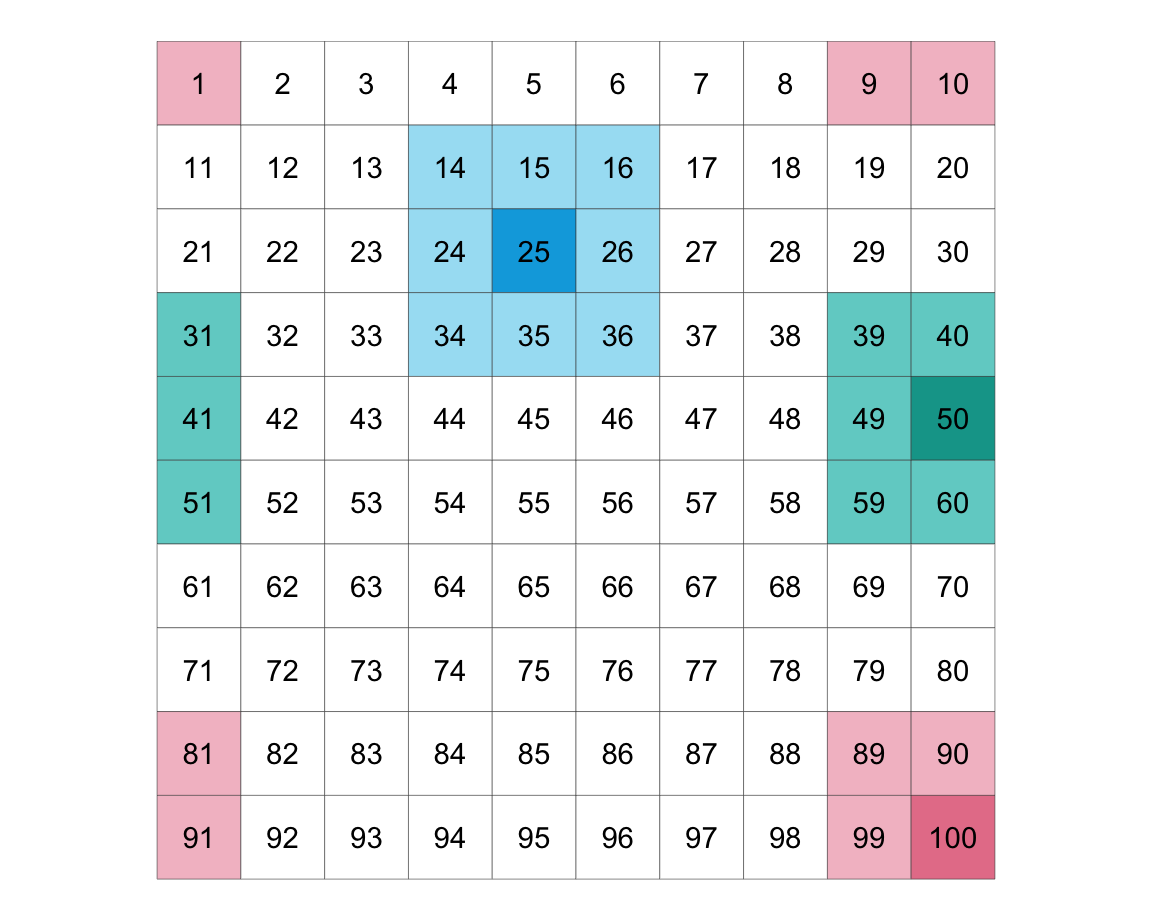Figure 17.1: Illustrating a torus structure of 100 individuals arranged on a 10x10 lattice.

Consequences:

The lattice/torus design creates a spatial continuum of agents, and strategies, and interactions. From the perspective of each individual agent, this setup multiplies the number of games she plays and the diversity of strategies she encounters. From the modeler’s perspective, this setup allows assessing the density of each strategy at each point in time, as well as the diffusion of strategies over time. Under some circumstances, a global pattern may emerge out of local interactions.

1. Some utility functions:
# exp_ceiling() limits the exponential of x
# to a maximum value of 2^1023:
exp_ceiling <- function(x) {

exp_x <- exp(x)  # compute exp once

ix_inf <- which(is.infinite(exp_x))  # ix of infinite values

if (length(ix_inf) == 0) {

return(exp_x)  # return as is

} else { # limit infinite values:

exp_x[ix_inf] <- 8.988466e+307  # 2^1023

return(exp_x)

}
}

## Check:
# x <- c(10, 100, 1000)  # Note: exp(710) => Inf
# exp(x)
# exp_ceiling(x)

# Simple vector calculation functions (to use in apply()):
divide_vec = function (num, den) {

return(num/den)

}

multiply_vec = function (Q, beta) {

return(Q * beta)

}
1. Set learner parameters:
# Learner:
epsilon   <- 0.1  # exploration rate (epsilon-greedy model)
alpha     <- 0.5  # learning rate/step size parameter
Q_initial <- 0    # initial payoff expectation
Q         <- array(dim = c(N_opt, N_t, N_p))
Q[ , 1, ] <- Q_initial

Note the 3-dimensional array of Q values (with dimensions of N_opt, N_t, and N_p, i.e., providing 2 x 100 x 100 slots).

1. Prepare data structures for storing intermediate results:
# Storing history:
choices       <- matrix(nrow = N_p, ncol = N_t)  # in wide format
payoffs       <- matrix(nrow = N_p, ncol = N_t)
performance   <- matrix(nrow = N_p, ncol = N_t)

choiceCounter   <- array(0, dim = c(N_opt, N_p))
riskyChoiceProb <- matrix(nrow = N_p, ncol = N_t)
netChoiceProb   <- array(dim = c(N_opt,  N_t, N_p))
netChoiceProb[ , 1, ] <- 1/N_opt

density_1 <- rep(NA, N_t)
density_2 <- rep(NA, N_t)

# this is a list where the tactics distribution of each time step will be recorded
all_choices <- list() 

#### Simulation

Running the simulation as a for loop (for each time step $$t$$):

for (t in 1:N_t) {

# Each individual chooses one option based on his/her choice probability netChoiceProb[ , t, ]:
choices[ , t] = apply(netChoiceProb[ , t, ], MARGIN = 2,
FUN = function(netChoiceProbInstance){
sample(1:N_opt, 1, prob = netChoiceProbInstance, replace = FALSE)})

all_choices$step[[t]] <- matrix(choices[ , t], nrow = LAT, ncol = LAT, byrow = TRUE) # Calculate the density of each option: density_1[t] <- which(choices[ , t] == 1) %>% length() %>% divide_vec(., LAT^2) density_2[t] <- which(choices[ , t] == 2) %>% length() %>% divide_vec(., LAT^2) # OR: # density_2[t] <- 1 - density_1[t] # Calculate payoffs of each player: # Assumption: Each player plays the game once against each of the 8 neighbors: for (p in 1:N_p) { # the position (address?) of the focal player in the lattice: i <- (p - 1) %/% LAT + 1 # quotient j <- (p - 1) %% LAT + 1 # remainder payoffs[p, t] <- 0 # initialize value for (g in c(-1, 0, 1)) { # neighbor x for (h in c(-1, 0, 1)) { # neighbor y if (g^2 + h^2 > 0) { # is FALSE iff g == h == 0, which eliminates the focal player: # Determine current payoffs (given player choices): cur_payoffs <- get_payoffs(opt_p1 = all_choices$step[[t]][i, j],
opt_p2 = all_choices$step[[t]][boundary_cond(i+g), boundary_cond(j+h)]) cur_payoff_p1 <- cur_payoffs # payoff of focal player payoffs[p, t] <- payoffs[p, t] + cur_payoff_p1 # accumulate payoffs of focal player } # if. } # for h. } # for g. } # for p. # cur_choices tracks which option was chosen by each individual on this trial: cur_choices <- choices[ , t] + N_opt * (1:N_p - 1) # Updating Q values (only if another trial is following): if (t < N_t) { # Copying the previous Q value to the current Q value: Q[ , t + 1, ] <- Q[ , t, ] QQ <- aperm(Q, c(1, 3, 2)) # transpose dimensions 2 and 3 of Q dim(QQ) <- c(N_opt * N_p, N_t) # Q value updating (note: alpha is a step-size parameter which is 1/n; the sample averaging rule): QQ[cur_choices, t + 1] <- QQ[cur_choices, t] + alpha * (payoffs[ , t] - QQ[cur_choices, t]) dim(QQ) <- c(N_opt, N_p, N_t) Q <- aperm(QQ, c(1, 3, 2)) ############### ## Softmax choice (based only on Q values): ############### Q_exp <- (Q[ , t + 1, ] * rep(20, each = N_opt)) %>% apply(., MARGIN = 2, FUN = exp_ceiling) %>% round(., 5) softmaxMatrix <- Q_exp %>% apply(1, divide_vec, den = apply(Q_exp, MARGIN = 2, FUN = sum)) %>% t() %>% round(., 5) ############### ## Softmax end. ############### ## Update choice probability matrix: netMatrix <- apply(X = softmaxMatrix, MARGIN = 1, FUN = multiply_vec, beta = (1 - epsilon)) %>% t() + apply(matrix(rep(1/N_opt, N_opt * N_p), N_opt:N_p), MARGIN = 1, FUN = multiply_vec, beta = epsilon) %>% t() %>% round(., 4) netChoiceProbAperm <- aperm(netChoiceProb, c(1, 3, 2)) dim(netChoiceProbAperm) <- c(N_opt * N_p, N_t) dim(netMatrix) <- c(N_opt * N_p, 1) netChoiceProbAperm[ , t + 1] <- netMatrix dim(netChoiceProbAperm) <- c(N_opt, N_p, N_t) netChoiceProb <- aperm(netChoiceProbAperm, c(1, 3, 2)) } # if (t < N_t). } # for t. Note some assumptions: • Each player selects her choice once at the beginning of each trial. Thus, it does not consider its opponents individually. #### Results Visualizing results for a population of agents requires calculating the density of their choices, rather than individual ones. This is why we computed the density_1 and density_1 vectors during the simulation: • Density dynamics of option choices over 100 trials: # Prepare data frame: density_data <- data.frame(t = 1:N_t, density_1 = density_1, density_2 = density_2) density_long <- density_data %>% pivot_longer(cols = c(-t), names_to = 'option', values_to = 'density') ggplot(density_long) + geom_hline(yintercept = 1/N_opt, linetype = 'dashed', color = "grey50")+ geom_line(aes(t, density, color = option), size = 1, alpha = 3/4)+ scale_color_manual(values = c('density_1' = usecol(Pinky), 'density_2' = usecol(Seeblau)), labels = c("Option 1", "Option 2")) + ylim(c(0, 1)) + labs(title = "Density dynamics of options over time", x = "Time step t", y = "Option density", color = "Options:") + theme_ds4psy()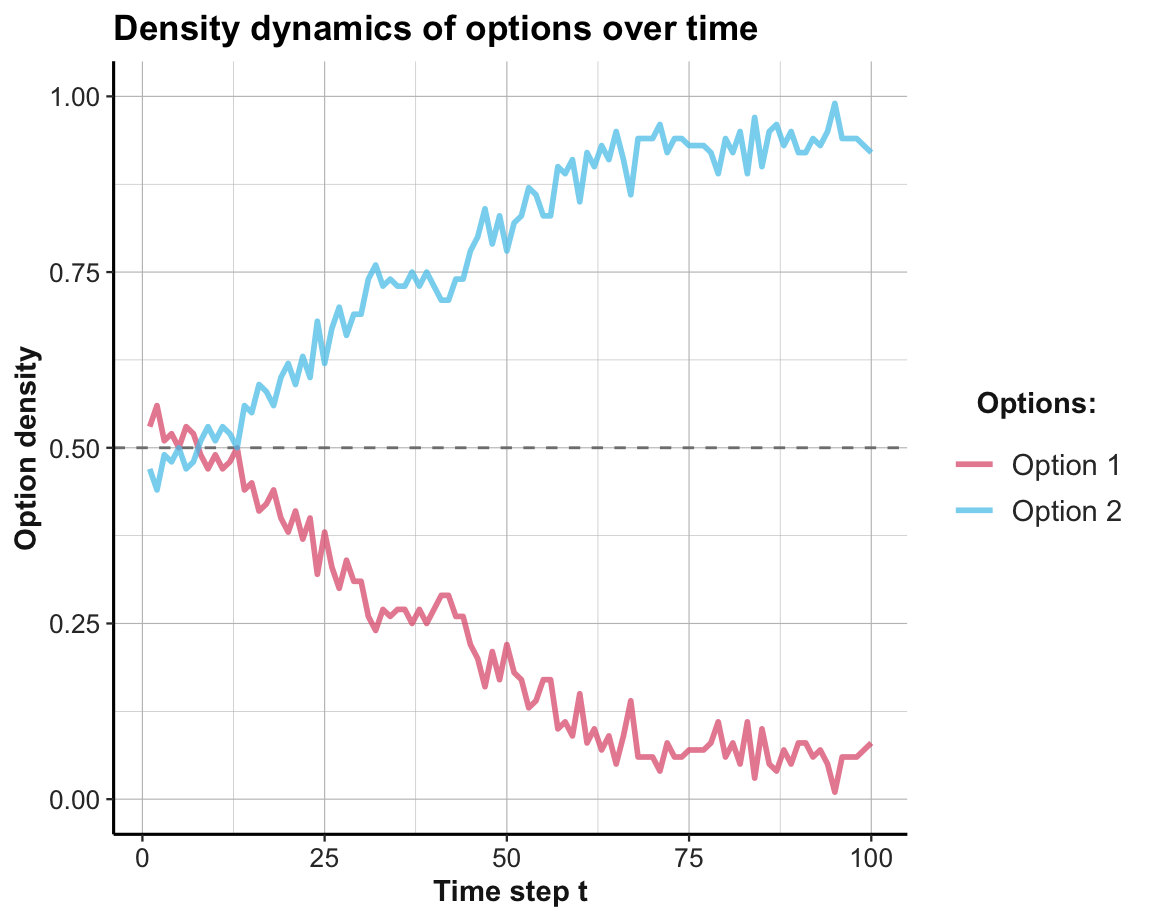#### Creating an animated plot To visualize the spatical dynamics of agent choices over time, we can show which opten each agent on the lattice/torus has chosen on every trial. This can be visualized as an animated gif, in two steps: • Prepare data: Transform the 3-dimensional array of all_choices into a 2-dimensional table all_choices_tb for (t in 1:N_t) { matrix <- as_tibble(all_choices$step[[t]], .name_repair = "unique")
names(matrix) <- as.character(1:LAT)
matrix$y <- 1:LAT if (t == 1) { all_choices_tb <- matrix %>% pivot_longer(cols = c(-y), names_to = 'x', values_to = 'option') all_choices_tb$x <- as.numeric(all_choices_tb$x) all_choices_tb$trial <- rep(t, LAT^2)

} else {

all_choices_tb_temp <- matrix %>%
pivot_longer(cols = c(-y), names_to = 'x', values_to = 'option')
all_choices_tb_temp$x <- as.numeric(all_choices_tb_temp$x)
all_choices_tb_temp\$trial <- rep(t, LAT^2)

all_choices_tb <- rbind(all_choices_tb, all_choices_tb_temp)

} # if.
}

# Check result:
dim(all_choices_tb)
#>  10000     4
#> # A tibble: 6 x 4
#>       y     x option trial
#>   <int> <dbl>  <int> <int>
#> 1     1     1      2     1
#> 2     1     2      2     1
#> 3     1     3      1     1
#> 4     1     4      1     1
#> 5     1     5      2     1
#> 6     1     6      2     1
• Create an animated image (by using the gganimate and gifski packages):
library(gganimate)
library(gifski)

animate_choices <- all_choices_tb %>%
ggplot() +
geom_raster(aes(x, y, fill = as.factor(option)))+
scale_fill_manual(values = c('1' = pal_pinky[], '2' = pal_seeblau[]),
labels = c("Option 1", "Option 2")) +
scale_x_continuous(breaks = 1:10, labels = 1:10) +
scale_y_continuous(breaks = 1:10, labels = 1:10) +
coord_equal() +
theme_ds4psy() +
# gganimate-specific parts:
transition_time(trial) +
labs(title = "Trial {frame_time} of a coordination game",
fill = "Options:", x = "", y = "") +
ease_aes("linear")

animate(animate_choices, duration = 10, fps = 10,
width = 400, height = 400, renderer = gifski_renderer())

# anim_save("images/soc_anim_coord.gif")  # save image

The resulting Figure 17.2 shows that both options are equally prominent at first, but the superior Option 2 is becoming more popular from Trial 10 onwards. Due to the agents’ tendency to explore, Option 1 is still occasionally chosen.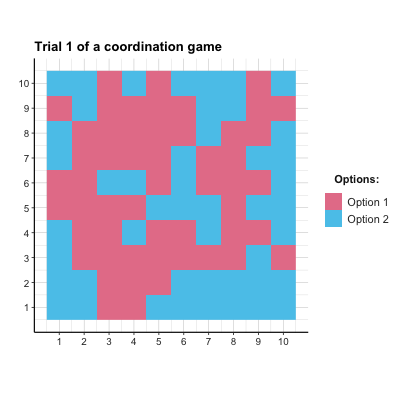Figure 17.2: The distribution of agent choices over time in the coordination game.

#### Practice

This exercise adjusts the simulation of the coordination game to the matching pennies (MP) game (defined by Table 17.1):

1. What is the optimal strategy that agents should learn in a purely competitive game?

2. What needs to be changed to use the above model to simulate this game?

#### Solution

• ad 1.: As any predictable agent could be exploited, the optimal choice for an individual agent is to randomly choose options. However, it is unclear what this would mean for a population of agents arranged on a lattice/torus.

• ad 2.: Theoretically, we only need to re-define the payoff matrix of the game (shown in Table 17.1). However, as the model above yields an error (when converting negative payoffs into probabilities), we add 1 to all payoffs. This turns a zero-sum game into a competitive game with symmetrical payoffs, but has no effect on the absolute differences in payoffs that matter for our RL agents):

# Payoff matrix (per player) of a competitive (matching pennies, MP) game:
payoff_p1 <- matrix(c(1, -1, -1, 1), nrow = N_opt, byrow = TRUE) + 1  # Player 1
payoff_p2 <- matrix(c(-1, 1, 1, -1), nrow = N_opt, byrow = TRUE) + 1  # Player 2
• ad 3.: Figure 17.3 shows the result of a corresponding simulation. In this particular simulation, a slight majority of agents chose Option 1, but the population did not reach a stable equilibrium. As each agent’s optimal strategy is to choose options randomly (i.e., with a $$p=.5$$ for each option), this could be evidence for learning. But to really demonstrate successful learning, further examinations would be necessary.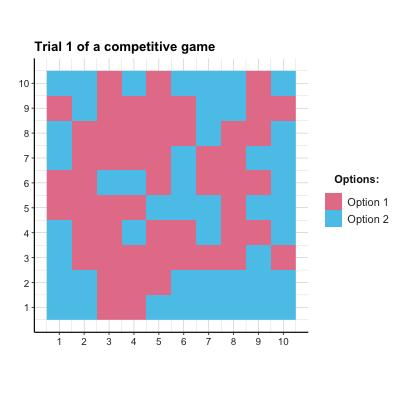Figure 17.3: The distribution of agent choices over time in a competitive game.

### 17.2.2 Social learning

Learning can be modeled as a function of rewards and the behavior of others. When an option is better than another, it offers higher rewards and will be learned by an agent that explores and exploits an environment to maximize her utility. However, when other agents are present, a second indicator of an option’s quality is its popularity: Other things being equal, better options are more popular.19

The basic idea of replicator dynamics (following Page, 2018, p. 308ff.) is simple: The probability of choosing an action is the product of its reward and its popularity.

Given a set of $$N_opt$$ alternatives with corresponding rewards $$\pi(1) ... \pi(n)$$, the probability of choosing an option $$k$$ at time step $$t+1$$ is defined as:

$P_{t+1}(k) = P_{t}(k) \cdot \frac{\pi(k)}{\bar{\pi_{t}}}$

Note that the factor given by the fraction $$\frac{\pi(k)}{\bar{\pi_{t}}}$$ divides the option’s current reward by the current average reward of all options. Its denominator is computed as the sum of all reward values weighted by their probability in the current population. As more popular options are weighted more heavily, this factor combines an effect of reward with an effect of popularity or conformity. Thus, the probability of choosing an option on the next decision cycle depends on its current probability, its current reward, and its current popularity.

Note that this particular conceptualization provides a model for an entire population, rather than any individual element of it. Additionally, the rewards received from each option are assumed to be fixed and independent of the choices, which may be pretty implausible for many real environments.

What changes would signal that the population is learning? The probability distribution of actions being chosen in each time step.

#### Implementation in R

# Environment parameters:
alt  <- c("A", "B", "C")
rew  <- c(20, 10, 5)
prob <- c(.1, .7, .2)  # initial probability distribution
N_t  <- 10  # number of time steps/rounds/trials

# Prepare data storage:
data <- as.data.frame(matrix(NA, ncol = 5, nrow = 10 + 1))
names(data) <- c("t", "avg_rew", paste0("p_", alt))

for (t in 0:N_t){

# (1) Compute average reward:
avg_rew <- sum(prob * rew)

# (+) User feedback/data storage:
# print(paste0(t, ": avg_rew = ", round(avg_rew, 1),
#              ": prob = ", paste0(round(prob, 2), collapse = ":")))
data[(t + 1), ] <- c(t, round(avg_rew, 2), round(prob, 3))

# (2) Update probability:
prob <- prob * rew/avg_rew

}

Given that we collected all intermediate values in data, we can inspect our simulation results by printing the table:

Table 17.3: Data from replicator dynamics.
t avg_rew p_A p_B p_C
0 10.00 0.100 0.700 0.200
1 11.50 0.200 0.700 0.100
2 13.26 0.348 0.609 0.043
3 15.16 0.525 0.459 0.016
4 16.89 0.692 0.303 0.005
5 18.18 0.819 0.179 0.002
6 19.01 0.901 0.099 0.000
7 19.48 0.948 0.052 0.000
8 19.73 0.973 0.027 0.000
9 19.87 0.987 0.013 0.000
10 19.93 0.993 0.007 0.000

As shown in Table 17.3, we initialized our loop at a value of t = 0. This allowed us to include the original situation (prior to any updating of prob) as the first line (i.e., in row data[(t + 1), ]).

Inspecting the rows of Table 17.3 makes it clear that the probabilities of suboptimal options (here: Options B and C) are decreasing, while the probability of choosing the best option (A) is increasing. Thus, options with higher rewards are becoming more popular — and the population quickly converges on choosing the best option.

The population’s systematic shift from poorer to richer options also implies that the value of the average reward (here: avg_rew) is monotonically increasing and approaching the value of the best option. Thus, the function of avg_rew is similar to the role of an aspiration level $$A$$ in reinforcement learning models (see Section 16.2.1), with the difference that avg_rew reflects the average aspiration of the entire population, whereas $$A_{i}$$ denotes the aspiration level of an individual agent $$i$$.

#### Visualizing results

Ways of depicting the shift in collective dynamics away from bad and towards the best option is provided by the following visualizations of data. Figure 17.4 shows the trends in choosing each option as a function fo the time steps 0 to 10:

library(tidyverse)
library(ds4psy)
library(unikn)

# Re-format data:
data_long <- data %>%
pivot_longer(cols = starts_with("p_"),
names_to = "option",
values_to = "p") %>%
mutate(opt = substr(option, 3, 3))
# data_long

ggplot(data_long, aes(x = t, y = p, group = opt, col = opt)) +
geom_line(size = 1.5, alpha = .5) +
geom_point(aes(shape = opt), size = 2) +
scale_y_continuous(labels = scales::percent_format()) +
scale_x_continuous(breaks = 0:10, labels = 0:10) +
scale_color_manual(values = usecol(c(Seegruen, Seeblau, Pinky))) +
labs(title = paste0("Probability trends over ", N_t, " steps"),
x = "Time steps", y = "Probability",
col = "Option:", shape = "Option:") +
theme_ds4psy()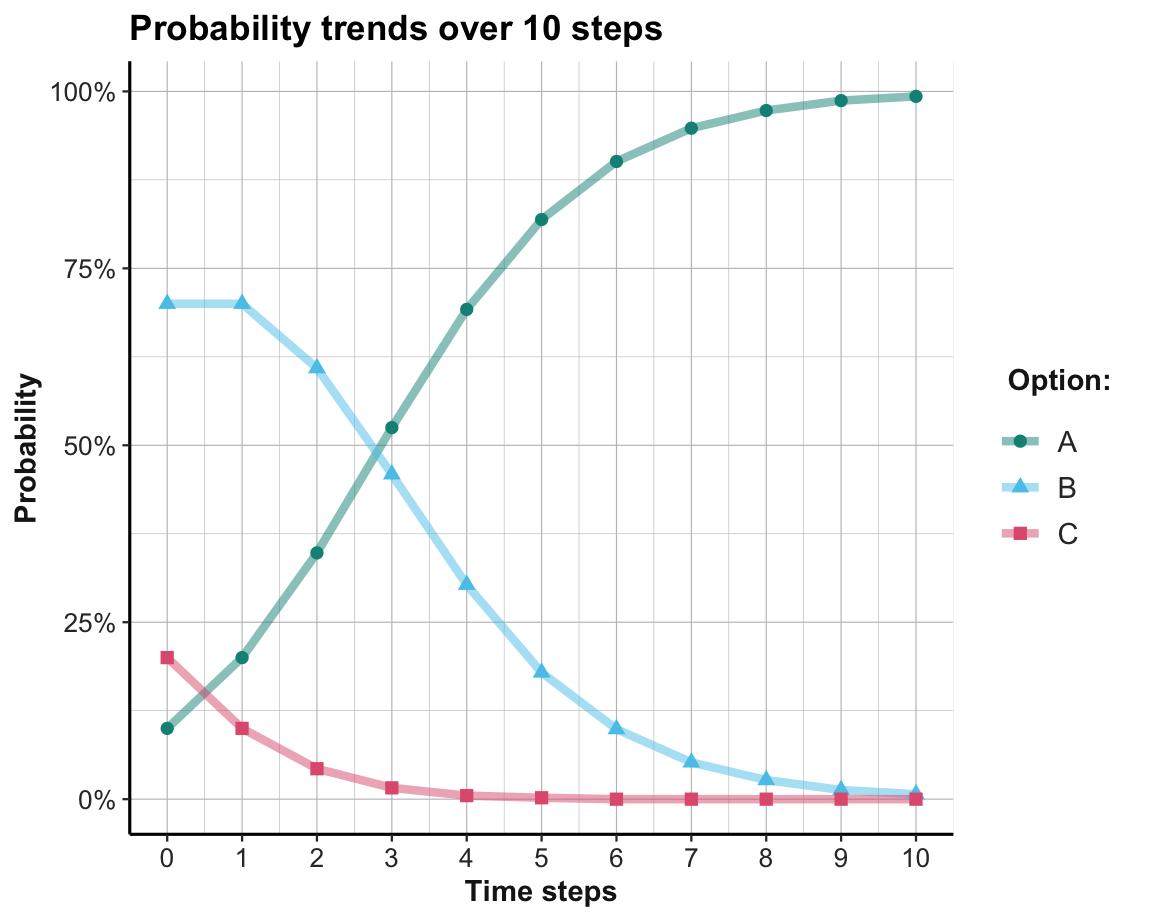Figure 17.4: Trends in the probabilty of choosing each option per time step.

Note that we re-formatted data into long format prior to plotting (to get the options as one variable, rather than as three separate variables) and changed the y-axis to a percentage scale.

Given that the probability distribution at each time step must sum to 1, it makes sense to display them as a stacked bar chart, with different colors for each option (see Figure 17.5):

ggplot(data_long, aes(x = t, y = p, fill = opt)) +
geom_bar(position = "fill", stat = "identity") +
scale_y_continuous(labels = scales::percent_format()) +
scale_x_continuous(breaks = 0:10, labels = 0:10) +
scale_fill_manual(values = usecol(c(Seegruen, Seeblau, Pinky))) +
labs(title = paste0("Probability distributions over ", N_t, " steps"),
x = "Time steps", y = "Probability",
col = "Option:", fill = "Option:") +
theme_ds4psy()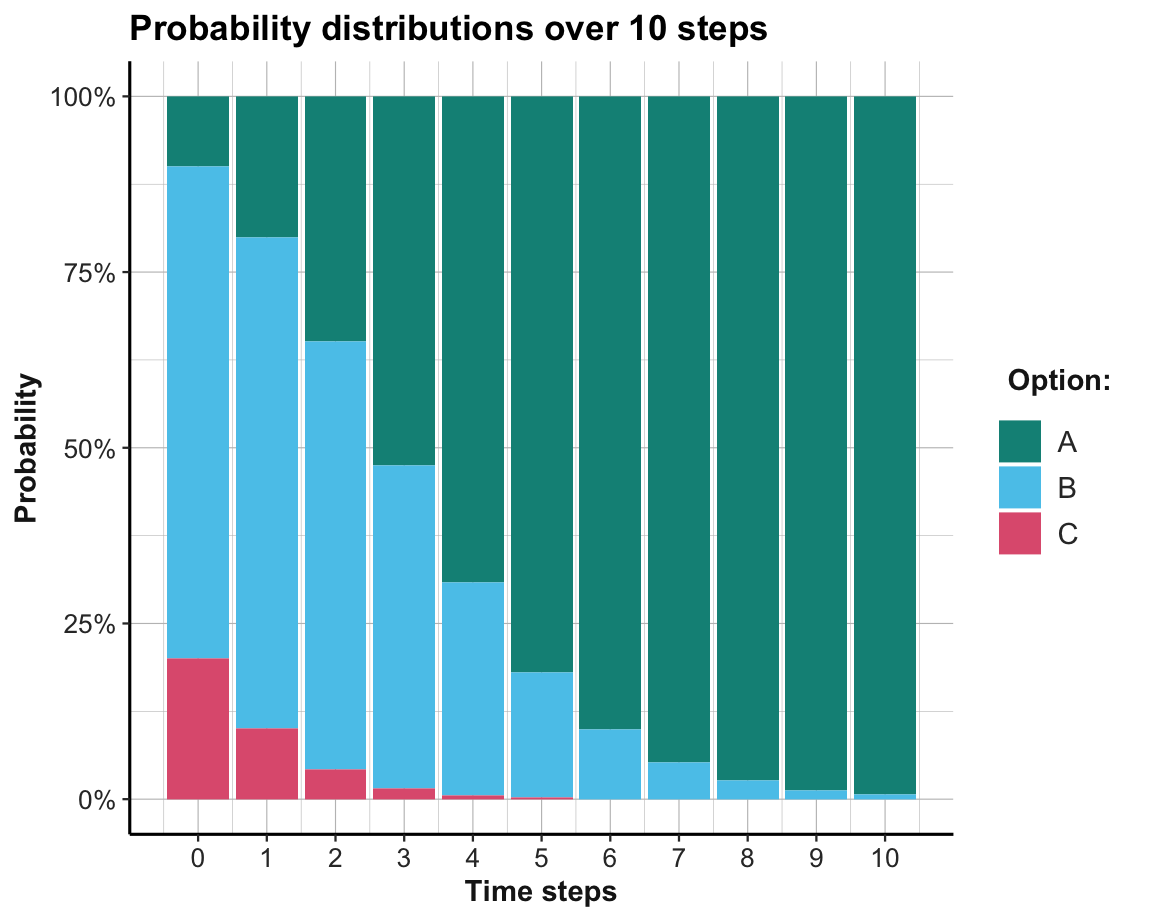Figure 17.5: The probabilty of choosing options per time step.

This shows that the best option (here: Option A) is unpopular at first, but becomes the dominant one by about the 4-th time step. The learning process shown here appears to be much quicker than that of an individual reinforcement learner (in Section 16.2.1). This is mostly due to a change in our level of analysis: Our model of replicator dynamics describes an entire population of agents. In fact, our use of the probability distribution as a proxy for the popularity of options implicitly assumes that an infinite population of agents is experiencing the environment and both exploring and exploiting the full set of all options on every time step. Whereas an individual RL agent must first explore options and — if it gets unlucky — waste a lot of valuable time on inferior options, a population of agents can evaluate the entire option range and rapidly converge on the best option.

Convergence on the best option is guaranteed as long all options are chosen (i.e., have an initial probability of $$p_{t=0}(i)>0$$) and the population of agents is large (ideally infinite).

#### Practice

1. Answer the following questions by studying the basic equation of replicator dynamics:

• What’s the impact of options that offer zero rewards (i.e, $$\pi(k)=0$$)?

• What’s the impact of options that are not chosen (i.e, $$P_{t}(k)=0$$)?

2. What happens if the three options (A–C) yield identical rewards (e.g., 10 units for each option)?

3. What happens if the rewards of three options (A–C) yield rewards of 10, 20, and 70 units, but their initial popularities are reversed (i.e., 70%, 20%, 10%).

• Do 10 time steps still suffice to learn the best option?

• What if the initial contrast is even more extreme, with rewards of 1, 2, and 97 units, and initial probabilities of 97%, 2%, and 1%, respectively?

Hint: Run these simulations in your mind first, then check your predictions with the code above.

1. What real-world environment could qualify as

• one in which the rewards of all objects are stable and independent of the agents’ actions?

• one in which all agents also have identical preferences?

1. How would the learning process change

• if the number of agents was discrete (e.g., 10)?

• if the number of options exceeded the number of agents?

Hint: Consider the range of values that prob would and could take in both cases.

### 17.2.3 Social networks

1. The list of “other things” that are assumed to be equal here is pretty long (e.g., including some stability in options and preferences).↩︎